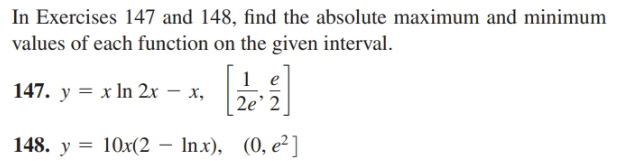# In Exercises 147 and 148, find the absolute maximum and minimum values of each function on the given interval. 147. y = x In 2x – x, 2e' 2 148. y = 10x(2 – Inx), (0, e²]

Questionhelp_outlineImage TranscriptioncloseIn Exercises 147 and 148, find the absolute maximum and minimum values of each function on the given interval. 147. y = x In 2x – x, 2e' 2 148. y = 10x(2 – Inx), (0, e²] fullscreen